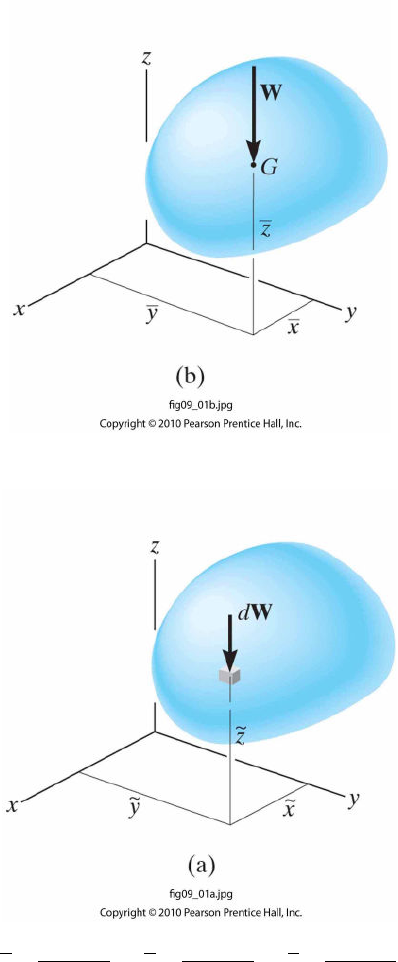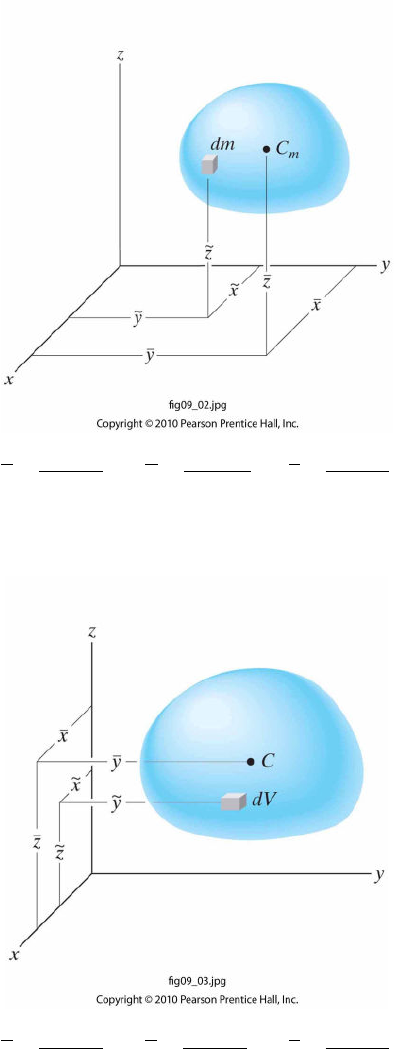Textbook Notes (270,000)
US (100,000)
LSU (20,000)
CE (800)
CE 2450 (100)
All (100)
Chapter

Student Handout Lesson 26Exam

Department
Civil Engineering
Course Code
CE 2450
Professor
All

This preview shows pages 1-2. to view the full 6 pages of the document.Chapter(9(
Center(of(Gravity(and(Centroid(
(
9.1(Center(of(Gravity,(Center(of(Mass,(and(the(Centroid(of(a(Body(
(
Center(of(Gravity!
!
To!determine!the!center!of!gravity!of!an!object!!
!
(
x =
˜
x dW
dW
(
y =˜
y dW
dW
(
z =
˜
z dW
dW
(
(

Only pages 1-2 are available for preview. Some parts have been intentionally blurred.Center(of(Mass(of(a(Body(
(
x =
˜
x d
m
dm
(
y =˜
y dm
dm
(
z =
˜
z dm
dm
(
(
Centroid(of(a(Volume(
(
x =
˜
x d
V
dV
(
y =˜
y d
V
dV
(
z =
˜
z dV
dV
(
(